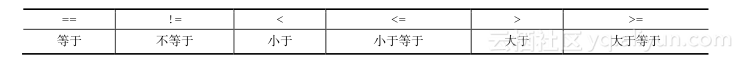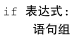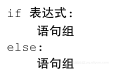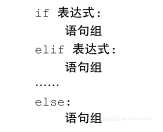《从问题到程序：用Python学编程和计算》——2.7　判断和条件控制

+关注继续查看

2.7.1　条件判断和逻辑表达式1）A与B，表示A和B都成立的组合判断；

2）A或B，表示A与B之中至少有一个成立的组合判断；

3）非A，表示A不成立的组合判断。

Python为上面三个逻辑组合关系提供了三个运算符：Python关于比较运算符和逻辑运算符的优先级和结合性的规定如下：

• 比较运算符的优先级低于算术运算符，因此x + y > x2相当于(x + y) > (x2)，是合法的关系表达式。所有比较运算符的优先级相同，连续的比较表示对相邻运算对象做关系运算之后用and连接，相互为“与”关系。
• 三个逻辑运算符中，一元运算符not的优先级最高，但低于比较运算符，or的优先级最低，and介于两者之间。因此，not x2 > 5 or y < 6 and x y > 8相当于not (x2 > 5) or ((y < 6) and (x y > 8))。

a > 0 and b > 0 and c > 0 and \
a + b > c and b + c > a and a + c > b

2.7.2　if语句（条件语句）# Program to calculate area of triangle
from math import sqrt

a = float(input("Length of edge a: "))
b = float(input("Length of edge b: "))
c = float(input("Length of edge c: "))

if a > 0 and b > 0 and c > 0 and \
a + b > c and b + c > a and a + c > b:
s = (a + b + c) / 2
area = sqrt(s * (s - a) * (s - b) * (s - c))
print("Area of the triangle:", area)
else:
print(a, b, c, "do not form a triangle!")

>>>
Length of edge a: 2
Length of edge b: 5
Length of edge c: 8
2.0 5.0 8.0 do not form a triangle

if语句的扩展形式求二次函数的根

from math import sqrt

print("Program for root(s) of quadratic equetions.")
a = float(input("Coefficient of x**2: "))
b = float(input("Coefficient of x: "))
c = float(input("Constant: "))

d = b**2 - 4 * a * c
if d > 0:
tmp = sqrt(d)
print("Two roots:", (-b + tmp)/2/a,  (-b - tmp)/2/a)
elif d == 0:
print("One root:", -b/2/a)
else:
print("No real root")

简单总计

# A program calculating total price of some goods

name = input("Please give the name of the good: ")
price = float(input("Price of one piece of the good: "))
amount = int(input("Amount: "))

if price > 0 and amount > 0:
print(amount, "pieces of", name,
"totally: ", price * amount)
else:
print("Error. Price and amount should be positive.")Python编程：Python2编码问题与pymysql查询结果乱码解决
Python编程：Python2编码问题与pymysql查询结果乱码解决
23 0easyUI中datagrid控制获取指定行数的数据

1221 0thinkphp-条件判断-范围判断-range标签
824 0UNIX环境高级编程笔记之进程控制
本章重点介绍了进程控制的几个函数：fork、exec族、_exit、wait和waitpid等，主要需要掌握的是父进程和子进程之间的运行机制，怎么处理进程的正常和异常终止、以及怎么让进程执行不同的程序等知识点。下一章将进一步说明一个进程和其他进程之间的关系——会话和作业控制。
678 0Python——条件判断
1,Python代码缩进规则: 具有相同缩进的代码视为代码块； 2,缩进请严格按照Python的习惯写法：4个空格，不要使用Tab，更不要混合Tab和空格，否则很容易造成因为缩进引起的语法错误。
803 0一些著名问题与算法 　　如果您的飞船破了一个洞，我只能深表同情，因为我所解决的99个问题里唯独没有这个问题。 　　——匿名者1 　　本文提到的所有问题与算法，因为有一些算法仅仅是为了试图说明某个原理，而有一些问题仅仅是为了某个算法而创造的。然而，作为索引，这里会列举出学习中最重要的那些问题与算法。 　　在本文大多数描述中，n代表的是问题规模，如一个序列中的元素数量。而在图论问题中，n表示的是节点的数量，m则表示边的数量。
13 0《Python高性能编程》——2.3　计算完整的Julia集合
3171 0552 0c++编程思想2 --友元存储控制
友元friend在c++中的应用  我们知道在c++的类访问权限中,private和 protected在类外面进行访问的时候 会因为权限而不能访问 ，友元就解决了这个问题 。 可以这样理解，他为外部的 函数 或者类 进行了 访问授权,其实这已经超出OOP的范畴,但是对于C++而言是以实用为主,所以说C++并不是完全面向对象的语言 C++这一特性就破坏的C++的安全性 。
707 0iOS：模态弹出窗控制器UIPopoverPresentationController
809 0
10059

0

+ 订阅

《2021云上架构与运维峰会演讲合集》

《零基础CSS入门教程》

《零基础HTML入门教程》